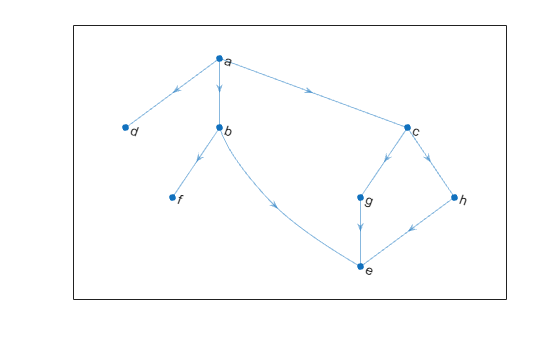Documentation

# predecessors

Node predecessors

## Syntax

``preIDs = predecessors(G,nodeID)``

## Description

example

````preIDs = predecessors(G,nodeID)` returns the predecessor nodes of the node in directed graph `G` specified by `nodeID`. The predecessor nodes form directed edges with `preIDs` as the source nodes, and `nodeID` as the target node.```

## Examples

collapse all

Create and plot a directed graph and determine the predecessor nodes of node `'e'`.

```s = [1 1 1 2 2 3 3 7 8]; t = [2 3 4 5 6 7 8 5 5]; names = {'a' 'b' 'c' 'd' 'e' 'f' 'g' 'h'}; G = digraph(s,t,[],names); plot(G)````preIDs = predecessors(G,'e')`
```preIDs = 3x1 cell array {'b'} {'g'} {'h'} ```

## Input Arguments

collapse all

Input graph, specified as a `digraph` object. Use `digraph` to create a directed graph object.

Example: `G = digraph([1 2],[2 3])`

Node identifier, specified as one of the values in this table.

ValueExample
Scalar node index`1`
Character vector node name`'A'`
String scalar node name`"A"`

Example: `preIDs = predecessors(G,3)` finds the predecessor nodes of node 3.

## Output Arguments

collapse all

Predecessor node IDs, returned as a node indices or node names.

• If `nodeID` is a numeric node index, then `preIDs` contains numeric node indices.

• If `nodeID` is a node name, then `preIDs` contains node names.

## Tips

• For multigraphs with multiple edges between the same two nodes, the output of `inedges` can be longer than that of `predecessors`, since there can be multiple incoming edges from some of the predecessors.